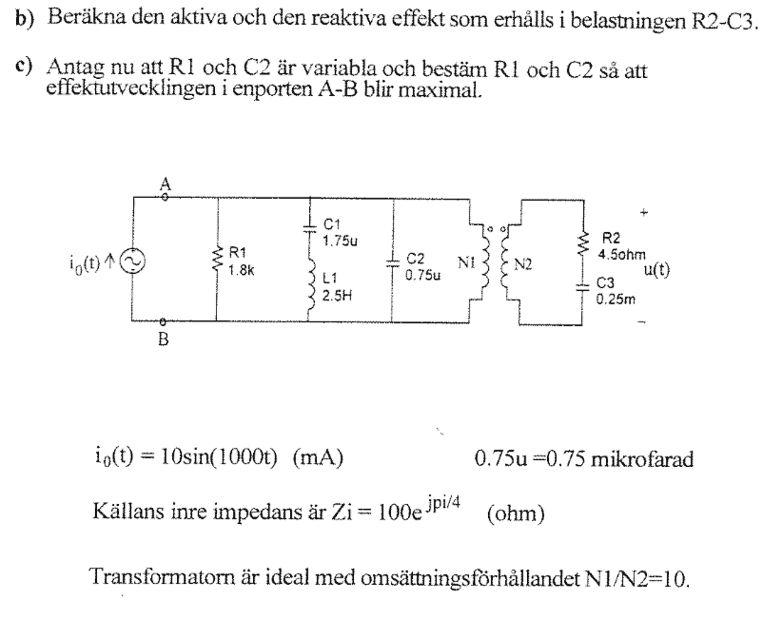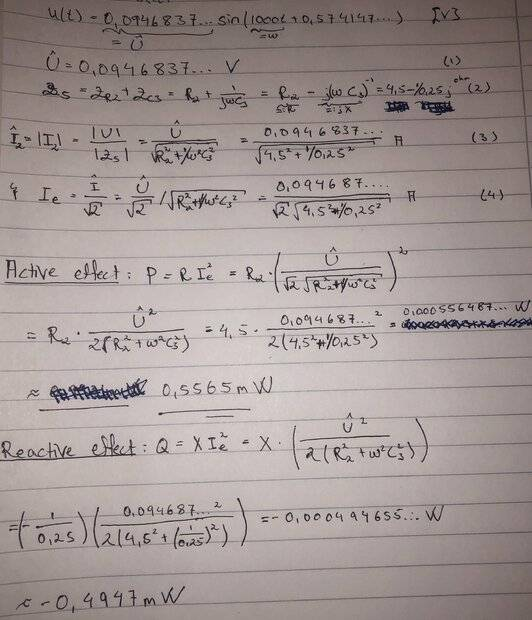# Active and reactive effect in AC circuit

• Engineering
• David331

#### David331

Homework Statement
Compute the active and reactive effect that is obtained in the load R2-C3.
Relevant Equations
P=RIe^2; Q=XIe^2
Hi, I am once again asking for help regarding a AC circuit problem. This time I need to compute reactive and active effect for z=R2+C3 in the below circuit. I have already computed u(t) from before so that solves half of the problem. I am unsure if I have done the calculations correct, not much if the number-computations are correct, but if the different formulas all make sense. And if the reactive effect can be negative?